# Guide For Processing PDE – Tutorial 1The best way to learn programming along with electronics is to interface as much as you can. Pocessing, is a simple language that lets you code animations and drawing and even graphics.

## Installing Processing PDE

• Visit : https://processing.org/

## Note to Linux Users

• Extract the ‘.tar’ file in the /opt directory. ( The Opt directory is for third party software packages )
• On the terminal type –
```saumitra@server-processor:~\$ cd /opt
saumitra@server-processor:/opt\$ cd processing-3.2.3
saumitra@server-processor:/opt/processing-3.2.3\$ ./processing```

This will launch the Processing PDE.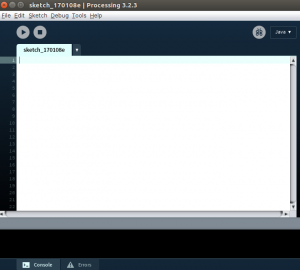## A Simple “Hi Sau” Program

Let us shift from typical “Hello World” convention to “Hi Sau” program (Sau = Extreme Short form of Saumitra) and we will continue to optimize the same piece of code further.

```void setup()
{
size(600,340);
smooth();
}

void draw()
{
if(mousePressed)
{
rect(30,20,4,60);
rect(30,50,50,4); // Print "H"
rect(80,20,4,60);

rect(120,20,4,60); // Prints "I"

rect(30,120,50,4);
rect(30,120,4,30);
rect(30,150,50,4); // Prints "S"
rect(80,150,4,30);
rect(80,180,-50,-4);

rect(120,120,4,60);
rect(120,120,30,4);
rect(150,120,4,60); //  Prints "A"
rect(120,150,30,4);

rect(180,120,4,60);
rect(180,180,40,4); //  Print "U"
rect(220,120,4,60);

}
}```

## Output

### No Mouse Press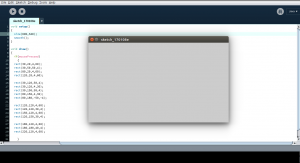Mouse Press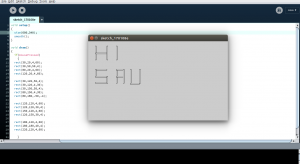## Detailed Explanation

• void : It is a keyword indicting that a function returns no value.
• setup( ) : This function runs once ( similar to Arduino IDE ), when the program runs. It defines critical properties like size or initial background conditions. There should be only one setup() function.
• size(x,y) : It defines the dimension of the display window width and height in unit of pixels. It should be inside the setup( ) function. Try replacing the size() function with ‘fullScreen( )’ which will print the output in full screen. The minimum x and y values 100 each in any direction.
• smooth( ) : Draws all geometry with smooth edges. This behavior is default so it could be removed. Use smooth( ) when there is a need of different style of smoothing. ‘smooth(level)’ , here level depends on the render value which will be used in the coming tutorials as required.
• draw( ) : It continuously executes the piece of code inside it similar to ‘loop( )’ in Arduino IDE. All Processing programs update the screen at the end of draw( ).
• mousePressed( ) : Stores whether or not a mouse button is currently pressed. As soon as mouse button is pressed, the condition becomes true and it then executed the block of code.
• rect(x, y, w, h) : Detailed discussion below.

## rect(x, y, w, h)

rect( ) draws a rectangle to the screen.

• x : Float- x coordinate of the rectangle. ( The x coordinate of the top left vertex by default )
• y : Float- y coordinate of the rectangle. ( The y coordinate of the top left vertex by default )
• w : Float- Width of  the rectangle.
• h : Float- Height of the rectangle.

Note : (0,0) is the coordinate of top left corner of the assigned screen.

## rect(x, y, w, h, a, tl, tr, br, bl )

• a : Radii for all four corners.
• tl : Radius for top-left corner.
• tr : Radius for top-right corner.
• br : Radius for bottom-right corner.
• bl : Radius for bottom-left corner.

### Example –

Case 1 : rect(30,30,200,205,20);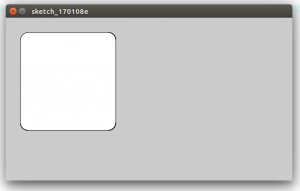Case 2 : rect(30,30,200,205,10,105,20,205);## rectMode( )

It modifies the location from which rectangles are drawn by changing the way parameters are given to rect( ). Let us understand in detail.

• rectMode(CORNER) : This is the default mode.
```void setup()
{
size(600,340);
smooth(255);
}

void draw()
{
rectMode(CORNER);
rect(30,30,50,50);
}```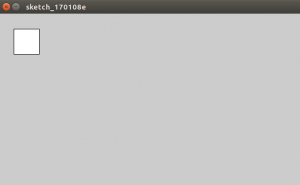In rect(30,30,50,50) , the rectangle is drawn with 30,30 being the x,y distance of the upper-left corner from 0,0.

• rectMode(CORNERS)
```void setup()
{
size(600,340);
smooth(255);
}

void draw()
{
rectMode(CORNERS);
rect(30,30,50,50);
}```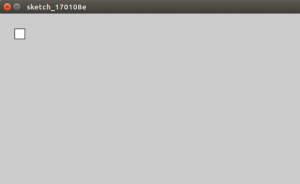Now, this will take first two parameter of rect( ) as the location of one corner and the last two parameters as the location of the opposite corner.

• rectMode(CENTER)
```void setup()
{
size(600,340);
smooth(255);
}

void draw()
{
rectMode(CENTER);
rect(25,25,50,50);
}```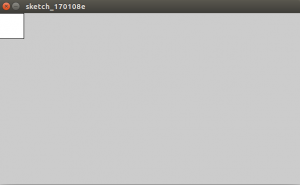It takes the first two parameters of rect( ) as the center point while the last two parameters as the width and height.

```void setup()
{
size(600,340);
smooth(255);
}

void draw()
{
rect(25,25,50,50);
}```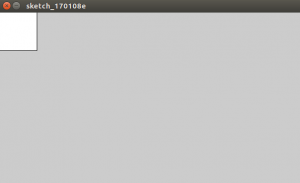This takes the first two parameters of rect( ) as the center point and the last two parameters as half of shapes’s width and height.

Enough with the boring content! Let us make a small fun code. Note : Processing can easily be integrated with Arduino and I’m going to do the same in the coming tutorials. Let us generate a typed letter on screen.

## See The Letter You Type

```void setup()
{
size(720,500);
textSize(120);
textAlign(CENTER);
}

void draw()
{
background(0);
text(key, 60, 80);
}```

## Output

When a is pressed :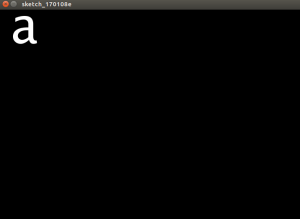## Detailed Explanation

• textSize( ) : Helps you to set the current font size. ( in pixels )
• text( ) : Draws text to the screen. Here the first parameter is key and the last two parameter indicate the X and Y coordinate.
• background( ) : Sets the color for background. Default is background is light gray. Here, we use as the rgb value. 255 sets the background value to white.
• textAlign( ) : Sets the alignment for the text. The current alignment is CENTER.